# Linear function facts for kids

Kids Encyclopedia Facts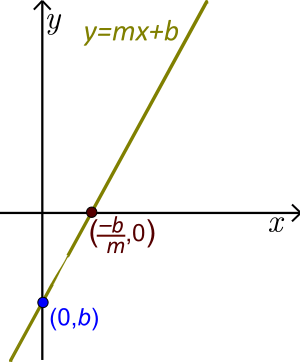Linear function (The line here is generic. It is slanted so m≠0. See examples with actual values for m and b below.)

In basic mathematics, a linear function is a function whose graph is a straight line in 2-dimensions (see images). An example is: y=2x–1. In higher mathematics, a linear function often refers to a linear mapping.

## Basic Properties

Formally, a linear function is a function f(x):RR such that the graph of f is a line. This means the domain or input of f is a real number R and the range or output of f is also a real number R. Usually we write y(x) or just y in place of f(x). So the formal statement means:

• we input or substitute a real number x into the linear function
• the linear function outputs or gives a real number y and
• all of these points (x,y) make a line.

There are three main forms for writing linear functions: slope-intercept, standard and parametric.

### Slope-intercept form

The slope-intercept (also called point-slope or explicit) form of a linear function is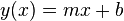$y(x)=mx+b$  or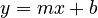$y=mx+b$ . This form has 2 variables x and у and 2 constants m and b.

• The letters m and b are constants. Before working with a linear function, we replace m and b with actual real numbers.
• The letters x and y are variables.
• The variable x is called the independent variable or argument. Any real number x can be input or substituted into a linear function. The function will then output the corresponding value for y.
• The variable y is called the dependent variable. It is the output value after substituting an input value for x.

• Horizontal lines are included. The line is horizontal if and only if m=0. Then we have just y=b. Since b is a real number, this is a constant function. So a constant function is also a linear function.
• Vertical lines are never included because a vertical line is not a function. A vertical line does not pass the vertical line test. (A vertical line is defined by the equation: x=b where b is a real number.)
• Slanted lines are included. The line is slanted if and only if m≠0.

• The slope-intercept form is unique. A different value of m or a different value of b gives a different line.
• A linear function is a polynomial function of first or zero degree in one variable х .
• The constant term is b. If we substitute x=0 into the function, we get y=b. So the number b is the y-intercept and the line crosses the у-axis at the point (0,b).
• If m≠0, the number –b/m is the x-intercept or root or zero and (–b/m,0) is the point where the line crosses the х-axis. Here the value of the function is zero.
• The coefficient m of x is called the slope or gradient of the line. For each line, the number m is a constant and so the slope is constant for the whole line. The slope determines both the direction and steepness of the line. Direction and steepness are called the rate of change. So the rate of change is m and it is constant for each line.
• The sign of m determines the direction. If m>0 then the linear function is increasing; if m<0 then the function is decreasing.
• The absolute value of m determines the steepness. If |m|<1 then the slope is gentle; if |m|>1 then the slope is steep.
• If the slope of a line is m and (х,у) is any point on the line, we must also have the point (х+1, y+m) on the line.

Example: y=–2x+4. The slope is m= –2 and the y-intercept is b=4 or the point (0,4). Substituting y=0 and solving for x, we get 0=–2x+4 or x=2. So x=2 is the root of this linear function and the point (2,0) is the x-intercept. Since the slope is m = –2, the line is decreasing. Since |–2|=2>1, the decrease is relatively steep. For each change in х of 1 (to the right), the value of у changes -2 (goes down).

• The graph of a line is determined by two points. To graph a linear function, we can substitute two different values for x into the function and solve for the corresponding y-values. We graph these two points. Using a straightedge, we draw the line through these two points extending it past both points.

Example: y=–2x+4. Substituting x=0 we get y=4 (this is the y-intercept) and thus the point (0,4). Substituting x=1, we get y=2 and thus the point (1,2). Plot these points and draw the line. (Notice that the 2nd point is 1 to the right and 2 down from the 1st point. As we said in the above example, this happens because the slope is m= –2)

• A linear function that is not a constant function is a bijection. It will output each real number (surjection) for exactly one input value (injection).

Example: y= –x+2. Suppose y= –1. We substitute y= –1 and get: –1= –'x+2 or x=3. This is the only solution. We can do this for any y-value.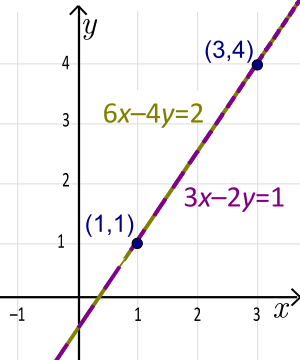Standard form of a line (non-unique). The unique slope-intercept form is y=1.5x–0.5

### Standard form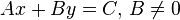$Ax+By=C , \, B \ne 0$.

• The standard form has 2 variables x and у and 3 constants A, B, and C that are replaced by actual real numbers before working. This form is often used in geometry and in systems of linear equations.

Example: The linear function 3x–2y=1 is in standard form. The constants are A=3, B=–2 and C=1.

• This form is sometimes written as: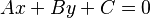$Ax+By+C=0$. So instead of writing 3x-2y=1, we write the equivalent equation 3x-2y-1=0.
• The constants A, B, and C are not uniquely determined. If we multiply them by a factor k, the value of these constants change, but the line is still the same.

Example: The lines 3x–2y=1 and 6x–4y=2 are coincident (same line). Here the factor is: k=2. We multiplied the first equation by 2 to get the second equation. The unique slope-intercept form of this line is: y=1.5x–0.5 (solve either equation for y).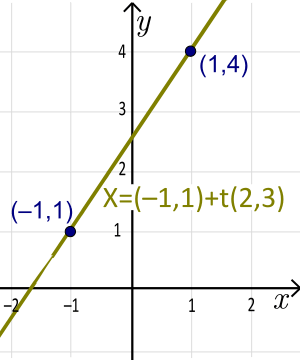Vector-parametric form of a line (non-unique). The unique slope-intercept form is y=1.5x+2.5

### Vector-Parametric form

Parametric form: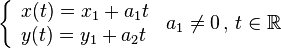$\left\{ {\begin{array}{*{20}{l}} {x(t) = {x_1}+{a_1}t }\\ {y(t) = {y_1}+{a_2}t } \end{array}} \right. \,{ a_1 \ne 0} \, ,\, t \in \mathbb{R}$    or

Vector form: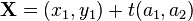${\mathbf{X}} = ({x_1},{y_1}) + t({a_1},{a_2})$  or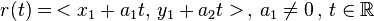$r(t)= \,< x_1+a_1 t, \, y_1 + a_2 t > \, , \,{ a_1 \ne 0} \, ,\, t \in \mathbb{R}$ .

• The parametric or vector or vector-parametric form has 1 parameter t, 2 variables x and у, and 4 constants a1, a2, x1, and y1. The coefficients a1, a2, x1, and y1 are not uniquely determined. The line passes through the points А=(x1,y1) and B=(x1+a1,y1+a2) so that taking taking any other points or even just reversing the order ot the points will result in different constants for the same line.
• The parameter t is not visible on the graph.
• Engineers usually use the letter t for the parameter. Mathematicians often use the Greek letter λ.

Example: X=(–1,1)+t(2,3), t∈R is a line in vector form. Here: a1=2, a2=3, x1=–1 and x2=1. The line goes through the points (x1,y1)=(–1,1) and (x1+a1,y1+a2)=(1,4). The corresponding parametric form of this line is: x(t)= –1+2t, y(t)=1+3t. The unique slope-intercept form of this line is: y(x)=1.5x+2.5   (solve the first equation for t and substitute this result into the second equation).

• The vector-parametric form of a line extends naturally to lines in 3-dimensional and higher spaces. The other forms do not.

Example: X=(–1,1,2)+t(2,3,–1), t∈R is a line in 3-dimensional space. The line goes through the points (–1,1,2) and (1,4,1).

## Derivative of a linear function

In the context where it is defined, the derivative of a function is a measure of the rate of change of function values with respect to change in input values. A linear function has a constant rate of change. This rate of change is the slope m. So m is the derivative. This is often written: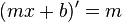$(mx+b)^\prime=m$

Example: y= –2x+4. Here m= –2 and so y′= –2.

## Linear function vs. Linear equation

Often, the terms linear equation and linear function are confused. Both are polynomials. However, the word linear in linear equation means that all terms with variables are first degree. (The word linear in linear function means the graph is a line.) A linear equation can have 1, 2, 3, or more variables. So a linear equation is a linear function only if it has exactly 2 variables. (A linear equation in one variable is a point on the number line and a linear equation in 3 variables is a plane in 3-dimensional space.)

## Notation

Many countries and disciplines use different letters and ordering for the different forms.

In many countries, a linear functions is often written as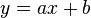$y=ax+b$ where a is the slope and b is the y-intercept.

In business and economics, a linear function is often written as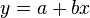$y=a+bx$ where a is the y-intercept and b is the slope.

## Related pagesLinear function Facts for Kids. Kiddle Encyclopedia.